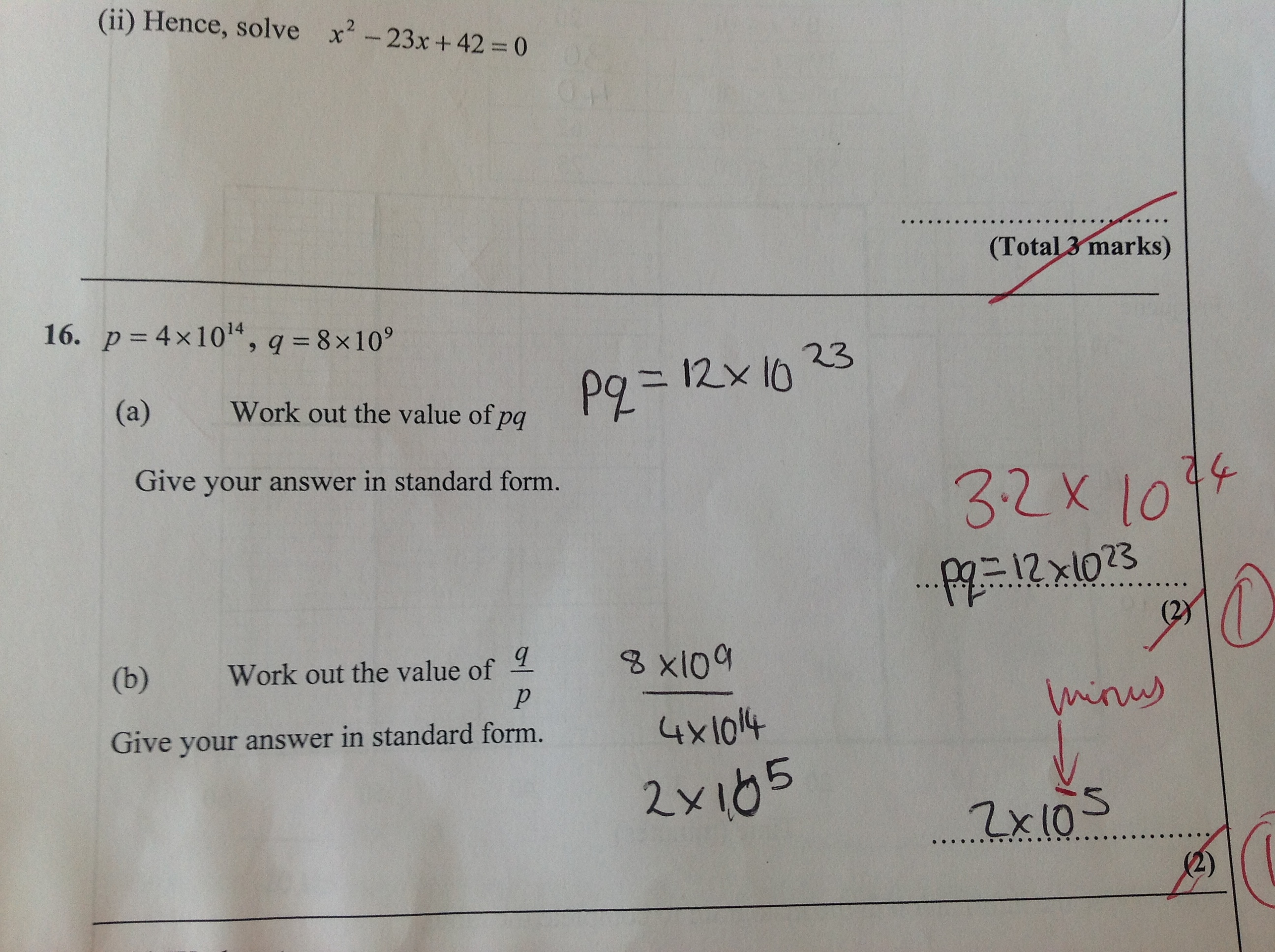Categories

# Scienfitic NotationWhat does the student know? What are the student’s misconceptions? How would you help?

## 4 replies on “Scienfitic Notation”

This student has not only memorized exponent rules but also tried to memorize what you do when you multiply two scientific notation statements.
If I were tutoring this student, I would have them talk me through how to multiply two numbers, say 6 x 3. I would have this student write this all out, explaining it to me like I did not know how to multiply. I would repeat this process with exponents. Then, I would have student explain to me what the question is asking. Hopefully they would see that this question is just multiplication and exponents, and be able to expand the problem to see what is really happening. We would do a few more where hopefully the student would discover the pattern they have been incorrectly trying to use, and see what they were trying to memorize is just a method to save time. Also, I would encourage the student to always expand when in doubt (or even if they just wanted to check their “shortcut magic” math work).

Jim Dohertysays:

What is curious to me here is that the work in part B looks stronger than the work in part A. I am used to seeing more difficulties with division. It seems clear to me that it is a simple subtraction mistake in part B although it could be that the subtraction was done in the wrong order. In part A, it seems that the new rule for multiplication (with exponents) has overwritten previous knowledge of multiplication. So, it used to be true that 4 X 8 = 32 but you have now told me to add when I see the multiplication symbol so 4 X 8 (when exponents are involved) REALLY means 4 + 8 now. I think it is important to constantly remind our students that we are expanding our math world view when we get new rules. we are not rewriting our math world rules. Consequently, I always try to use the word ‘yet’ in conversations. For example, early in the year in Alg II if there is a negative in a radical and someone tells me we cannot take the square root of a negative number, I say ‘yet’ at the end of their statement

louisesays:

Being cruel, I make the student write out all the tens. I make them do divisions with factors. Then do the division or multiplication. A student usually only has to do this once or twice, because I try and emphasize that math is strictly for the lazy, and if you know the exponent shortcut, you don’t have to do all that writing.

Part B looks suspiciously like what I see from students who “don’t like negatives.” Whenever a problem involves subtraction that results in a negative number, they simply flip the subtraction to keep things positive. Many of my 8th graders still struggled with negative numbers and would do anything they could (including making things up) to get rid of negative signs. My solution was to use negatives as much as possible in my classes, with the idea that familiarity would breed love for the negatives and respect for the non-commutativeness of subtraction.

For Part A, I agree with Jim and Julie. It looks like the student has overwritten their knowledge of integer multiplication in the process of learning how to multiply exponents. I usually solve this by having students write out their powers of ten and then multiply by hand a few times, looking for the pattern.